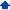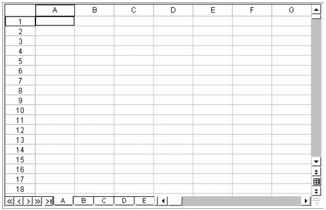### Understanding the components of a notebook

Notebooks, spreadsheets, and their associated elements are the core of the Quattro Pro application. Notebooks provide a way to organize many spreadsheets within the same file. There are 18,000 spreadsheets in a notebook. Each spreadsheet consists of approximately 1,000,000 rows and 18,000 columns.

#### Outline of notebook componentsThe following information describes the basic components of a Quattro Pro notebook.

A spreadsheet is an electronic ledger. It contains columns and rows in which you enter, arrange, calculate, and analyze data. In a spreadsheet, you can arrange and categorize data, perform simple math operations, and apply complex formulas. Once you enter your data in a spreadsheet, you can create a chart, add maps and graphics, or produce a report. The spreadsheet you see when Quattro Pro opens is one of thousands available in each notebook.##### Objects sheet

The last sheet of every notebook is the Objects sheet. The Objects sheet displays an icon for every chart in the notebook. This sheet also displays icons for custom dialog boxes you build. You can copy, rename, and print items in the Objects sheet. The Objects sheet property bar has buttons for creating, editing, and displaying charts and for building custom dialog boxes.

##### Project templates

Project templates let you create a new notebook based on a pre-designed project. Many of the Quattro Pro project templates provide a basic format and structure for common spreadsheets and data entry forms. You can also create your own project templates.

##### Values

A value is a number, date, formula, or the result of a formula. Quattro Pro automatically determines whether data is a value or a label. As you type your data in a cell, the READY indicator on the application bar changes to LABEL or VALUE, depending on the type of data you enter.

##### Labels

Labels contain alphanumeric data, such as titles, phone numbers, or addresses. Quattro Pro interprets and formats labels differently than it does values. Values are calculable; labels are not.

##### Formulas

Formulas are mathematical equations. Formulas usually refer to numbers in other cells in order to calculate a value, such as the difference between the values in two cells or the total of values in a column. You can use mathematical functions and numbers in formulas.